### LOWER Function Examples – Excel & Google Sheets

This tutorial demonstrates how to use the Excel LOWER Function in Excel to convert a text to lower case.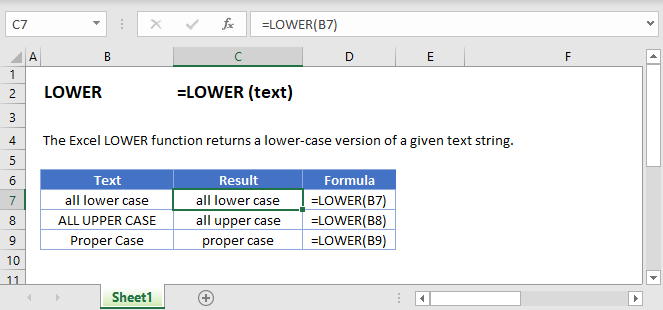## LOWER Function Overview

The LOWER Function Converts entire text string to lower case.

To use the LOWER Excel Worksheet Function, select a cell and type: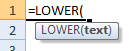(Notice how the formula inputs appear)

### LOWER function Syntax and inputs:

`=LOWER(text)`

text – A string of text.

AutoMacro - VBA Code Generator

## What Is the LOWER Function?

The Excel LOWER Function takes a string as an input, and returns same text string, but in lower case.

### How to Use the LOWER Function

Use the Excel LOWER function like this:

`=LOWER(“AUTOMATEEXCEL.COM”)`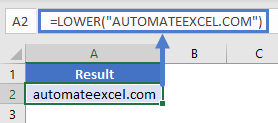This formula would return the following:

automateexcel.com

Here are a few more examples: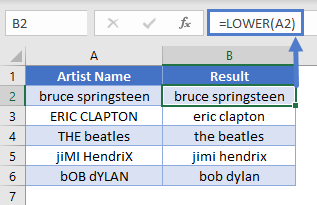## How LOWER Handles Numbers and Special Characters

The Excel LOWER Function has no effect on numbers, punctuation marks, or other special characters.

### How LOWER Handles Accented Letters

If you use LOWER on an upper-case accented letter, Excel will return its lower case accented equivalent wherever possible.

### Creating Email Addresses Based on Name Data

Imagine you have a list of contacts, and you want to add their email addresses to your spreadsheet. Because email addresses are usually presented in lower case, LOWER is an idea tool to use here.

To create the email addresses, we’d need to concatenate the first name, last name, the “@” email symbol, and the domain name. Concatenate basically just means “join together” – Excel will treat any strings connected by an “&” symbol as one single string.

When we’ve done that, we can just pass the entire concatenated string to LOWER, to get the lower-case version. Like this:

`=LOWER(A2&B2&"@"&C2)`

Here’s how it works: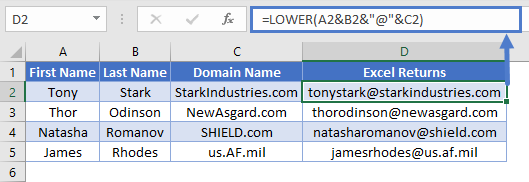Note that you can also use Excel’s CONCATENATE function for this. This function concatenates a comma-separated list of strings, like so:

`=LOWER(CONCATENATE(A2,B2,"@",C2))`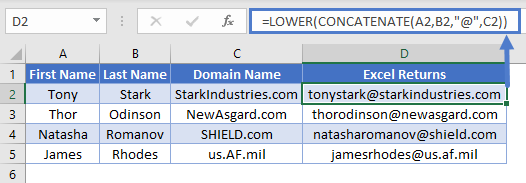## LOWER in Google Sheets

The LOWER Function works exactly the same in Google Sheets as in Excel: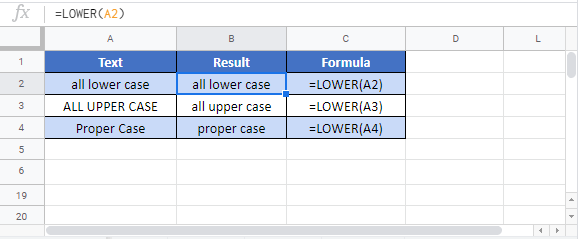## Additional Notes

The UPPER Function and LOWER Function are useful when working with case-sensitive Excel Functions such as Find Function and SUBSTITUTE Function. <>

Return to the List of all Functions in Excel

## Excel Practice Worksheet

Practice Excel functions and formulas with our 100% free practice worksheets!

• Automatically Graded Exercises
• Learn Excel, Inside Excel!